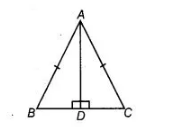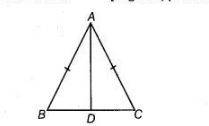# ABC is an isosceles triangle with AB = AC`
Question:

ABC is an isosceles triangle with AB = AC and D is a point on BC such that AD ⊥BC (see figure). To prove that ∠BAD = ∠CAD, a student proceeded as

followsIn $\triangle A B D$ and $\triangle A C D$, we have

$A B=A C$ [given]

$\angle B=\angle C$ [because $A B=A C$ ]

and $\angle A D B=\angle A D C$

Therefore, $\triangle A B D \cong \triangle A C D$ [by AAS congruence rule]

So, $\angle B A D=\angle C A D$ [by CPCT]

What is the defect in the above arguments?

Solution:

In $\triangle A B C$, $A B=A C$

$\Rightarrow \quad \angle A C B=\angle A B C \quad$ [angles oppsoite to the equal sides are equal]

In $\triangle A B D$ and $\triangle A C D$,$A B=A C$ [given]

$\angle A B D=\angle A C D$ [proved above]

$\angle A D B=\angle A D C$ [each 90°]

$\therefore$ $\triangle A B D \cong \triangle A C D$[by AAS]

So, $\angle B A D=\angle C A D$ [by CPCT]

So, the defect in the given argument is that firstly prove $\angle A B D=\angle A C D$

Hence, $\angle A B D=\angle A C D$ is defect.